# D H Conventions for Forward Kinematics

Abstract: This article mainly involves the difference between the D-H classical (or Distal) convention and D-H modified (or Proximal) convention for a kinematic chain. (a general abstract of the difference is to be finished...)

Keywords: D-H classical convention, D-H modified convention, forward kinematics

# Prerequisite Knowledge

## Normal

&quot;In geometry, a normal is an object such as a line or vector that is perpendicular to a given object. For example, in two dimensions, the normal line to a curve at a given point is the line perpendicular to the tangent line to the curve at the point."Rephrase "JK is perpendicular to AB" as:

• JK is normal to AB
• JK, AB meet at $90^\circ$
• JK, AB are at right angles

## Collinearlity

"In geometry, collinearity of a set of points is the property of their lying on a single line. A set of points with this property is said to be collinear (sometimes spelled as colinear). In greater generality, the term has been used for aligned objects."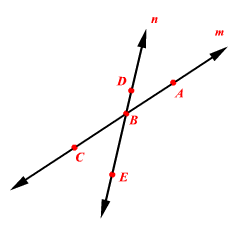# Forward Kinematics and Kinematic Chain

Forward kinematics seek to deduce the effect of the end-effector according to the variables of all joints. While inverse kinematics seek to calculate the rotation or translation of all joints based on the position and orientation of the end-effector.

Generally, this chapter introduces the basic knowledge of kinematic chain and illustrates the parameters of the chain, which leads to the significant link frame, the basis of D-H convention.

## Kinematic Chain

Generally, a kinematic chain, as a model of a robot serial-link manipulator, is a set of bodies, called links, connected by joints. The joints may be simply revolute joints or prismatic joints which have only one degree-of-freedom (dof) while they can also be quite complex with more than one dof. However, we tend to imagine all joints of a robot have only one dof because a complex joint is regarded as a combination of several single-dof joints, which make no difference to the analysis of the chain.

Reddy A. Chennakesava, Difference between Denavit-Hartenberg (DH) classical and modified conventions for forward kinematics of robots with case study

Note that the assumption does not involve any real loss of generality, since joints such as a ball and socket joint (two degrees-of-freedom) or a spherical wrist (three degrees-of-freedom) can always be thought of as a succession of single degree-of-freedom joints with links of length zero in between.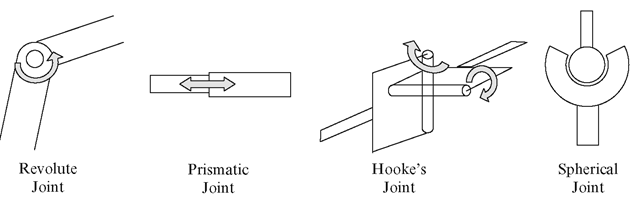To know more about joints, check: To be finished

Here comes the introduction of the specification of a manipulator using links and joints.

For a manipulator with n joints numbered from 1 to n, there are n+1 links, numbered from 0 to n. \begin{equation} \begin{aligned} &n,&joints:,,&1,...,n\\ &n+1,&links:,,&0,...,n \end{aligned} \end{equation} Link 0 is the base of the manipulator and often fixed.

Link n carries the end-effector. \begin{equation} \begin{aligned} link,i-1 \overset{\mbox{joint i}}\longleftrightarrow link,i \end{aligned} \end{equation} When joint i is actuated, link i moves.

To specify the elements, four parameters are used:

elementparametersdefinition
jointoffset lengthdistance from one link to the next along the axis of the joint
jointjoint anglerotation of one link with respect to the next about the joint axis

A coordinate frame (frame i) is attached rigidly to each link (link i).

Each joint has a joint axis. By convention, the z-axis of a coordinate frame is aligned with the joint axis.

A minimum of six dofs is required to describe an end-effector in space (position: 3 dof, orientation: 3 dof). To analyse a forward kinematic problem, we need the joint variables.

The displacement of joint is denoted by $q_i$ and is called joint variable. The collection of joint variables $q=[q_1,q_2,...,q_n]^T$ is called the joint vector. To define a point (the position of the end-effector), dimensional vector is used: $r=[e_1,r_2,...,r_m]^T$. The manipulator mechanism $r=f(q)$ defines the relation between r and q.

Links have a proximal end closest to the base and distal end closest to the tool. The proximal end of the link has the lower joint number. Each type of link has 4 parameters, 2 directions of translation and 2 axes of rotation.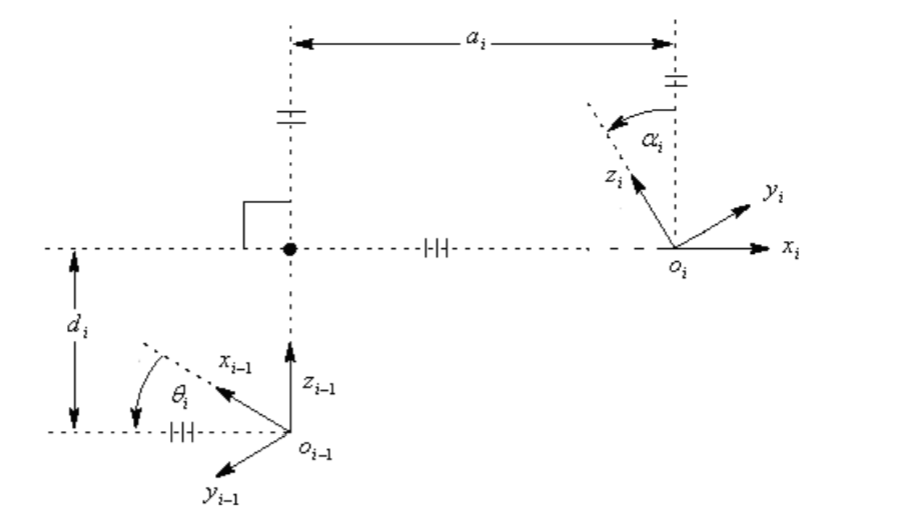Link parameters determine the structure of the link. In terms of size and shape between joint i and joint i+1:

• link length: $a_i$, distance measured along the common normal to both joint axes.

In robotics the common normal of two non-intersecting joint axes is a line perpendicular to both axes. When two consecutive joint axes are parallel, the common normal is not unique and an arbitrary common normal may be used, usually one that passes through the center of a coordinate system.

• twist angle: $\alpha_i$, the angle measured between the orthogonal projections of both joint axes onto a plane normal to the common normal.

It is a form of parallel projection, in which all the projection lines are orthogonal to the projection plane, resulting in every plane of the scene appearing in affine transformation on the viewing surface.

Joint parameters are the distance and angle between adjacent links and they determine the relative position of neighbouring links. In terms of relative displacement of the joint i:

• joint angle: $\theta_i$, rotation about the joint axis
• link offset: $d_i$, displacement along the joint axis

Define an coordinate frame $o_ix_iy_iz_i$attached to link i:

• z - axis: along the rotation direction for revolute joints, along the translation direction for prismatic joints
• $z_{i-1}$ axis lies along the axis of motion of the i-th joint
• origin $o_i$: intersection of joint axis $z_i$ with the common normal to $z_i$ and $z_{i-1}$
• $x_i$: along the common normal from joint i to joint i+1
• $y_i$: according to right-hand frame, $y_i=z_i\times x_i$

# Denavit-Hartenberg Conventions

The position and orientation of the end-effector is given by $$H=^0T_n=^0T_1^1T_2^2T_3...^{n-1}T_n$$

where, \begin{equation} \begin{aligned} ^{i-1}T_i= \left[ \begin{matrix} ^iR_{i-1}& ^id_{i-1}\\ 0 & 1 \end{matrix} \right] \end{aligned} \end{equation}

## Classical Convention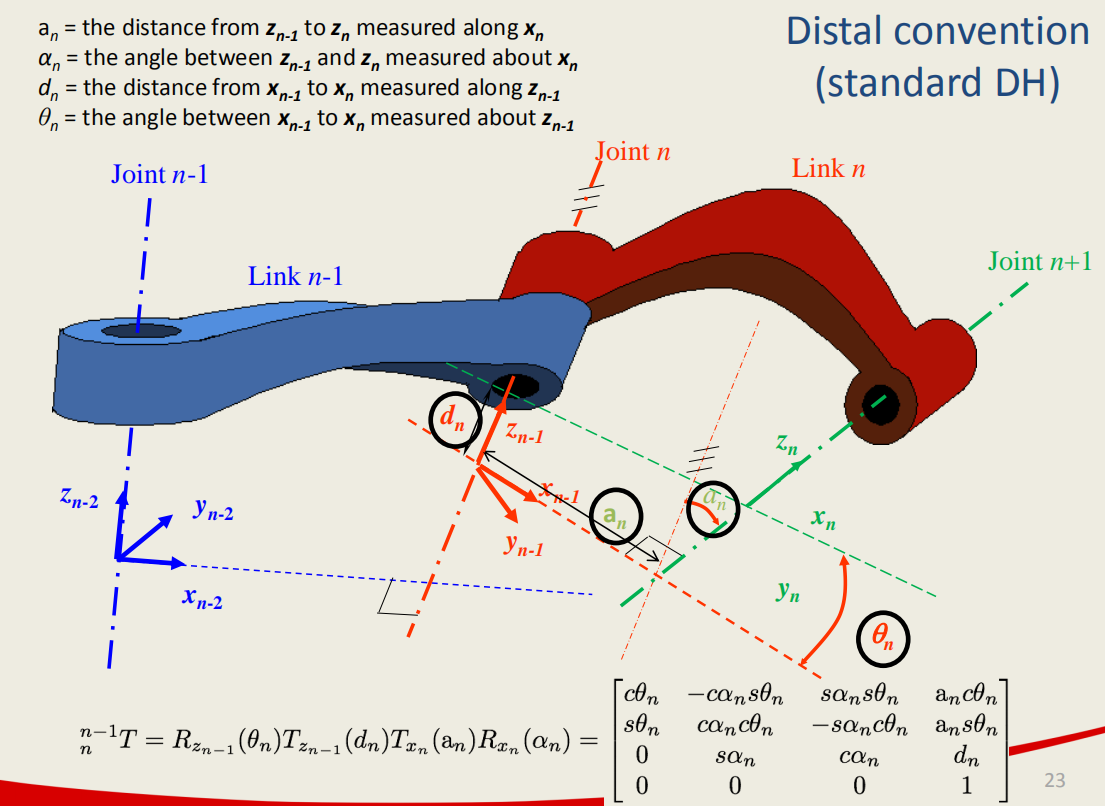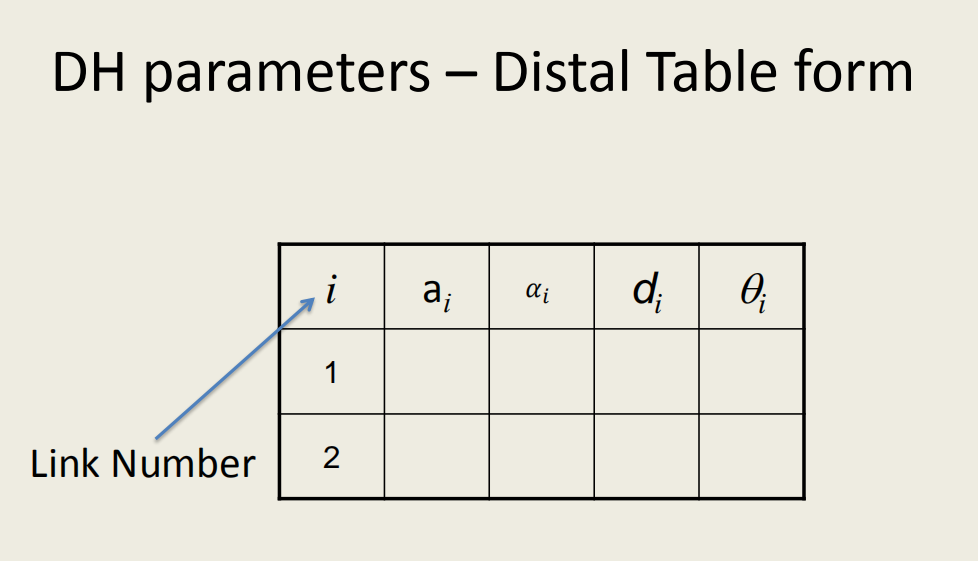The homogeneous transformation from link i-1 to link i is represented as a product of four basic transformations as follows: \begin{equation} \begin{aligned} ^{i-1}T_i&= R(z_{i-1},\theta_i)T(z_{i-1},d_i)T(x_i,a_i)R(x_i,\alpha_i)\\ &= \left[ \begin{matrix} cos\theta_i& -sin\theta_i &0 &0\\ sin\theta_i& cos\theta_i &0 &0\\ 0& 0& 0& 0\\ 0& 0& 0& 1 \end{matrix} \right] \left[ \begin{matrix} 1& 0 &0 &0\\ 0& 1 &0 &0\\ 0& 0& 1& d_i\\ 0& 0& 0& 1 \end{matrix} \right] \left[ \begin{matrix} 1& 0& 0& a_i\\ 0& 1& 0& 0\\ 0& 0& 1& 0\\ 0& 0& 0& 1 \end{matrix} \right] \left[ \begin{matrix} 1& 0 &0 &0\\ 0&sin\alpha_i& cos\alpha_i &0 \\ 0& cos\alpha_i& -sin\alpha_i& 0\\ 0& 0& 0& 1 \end{matrix} \right]\\ &= \left[ \begin{matrix} cos\theta_i& -cos\alpha_isin\theta_i& sin\alpha_isin\theta_i& a_icos\theta_i\\ sin\theta_i& cos\alpha_icos\theta_i& -sin\alpha_icos\theta_i& a_isin\theta_i\\ 0& sin\alpha_i& cos\alpha_i& d_i\\ 0& 0& 0& 1 \end{matrix} \right] \end{aligned} \end{equation}

## Modified Convention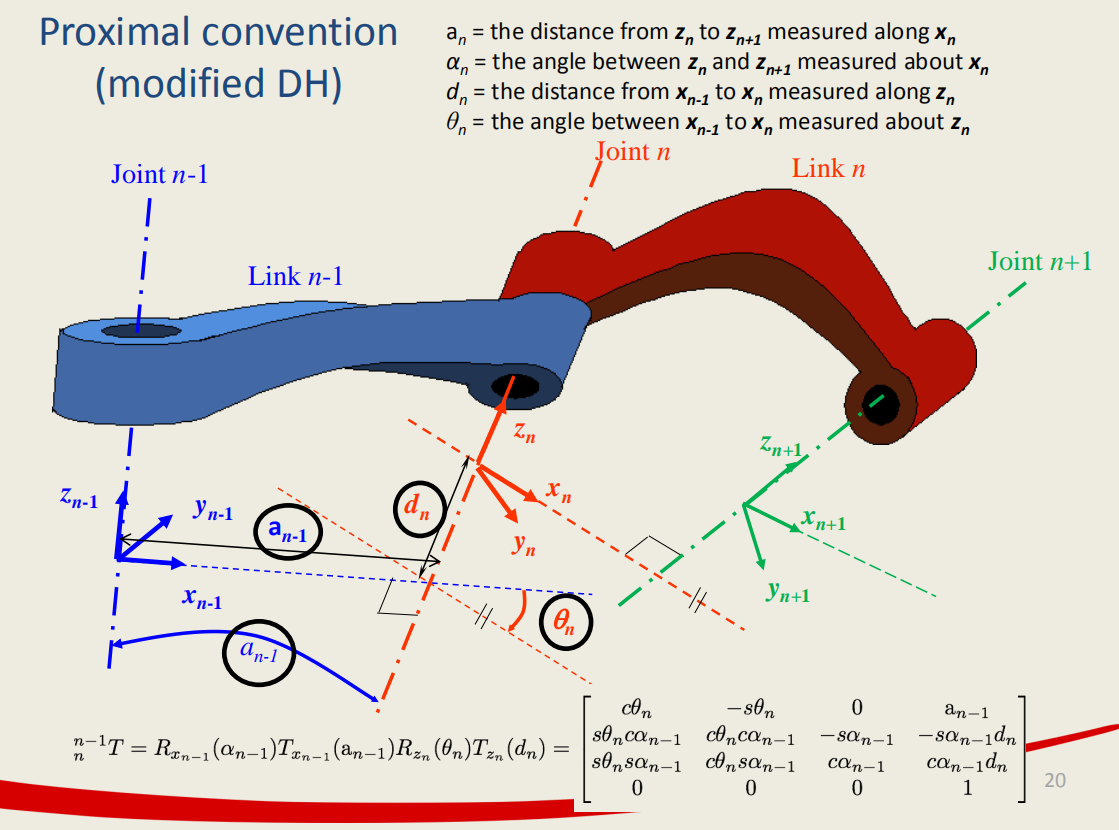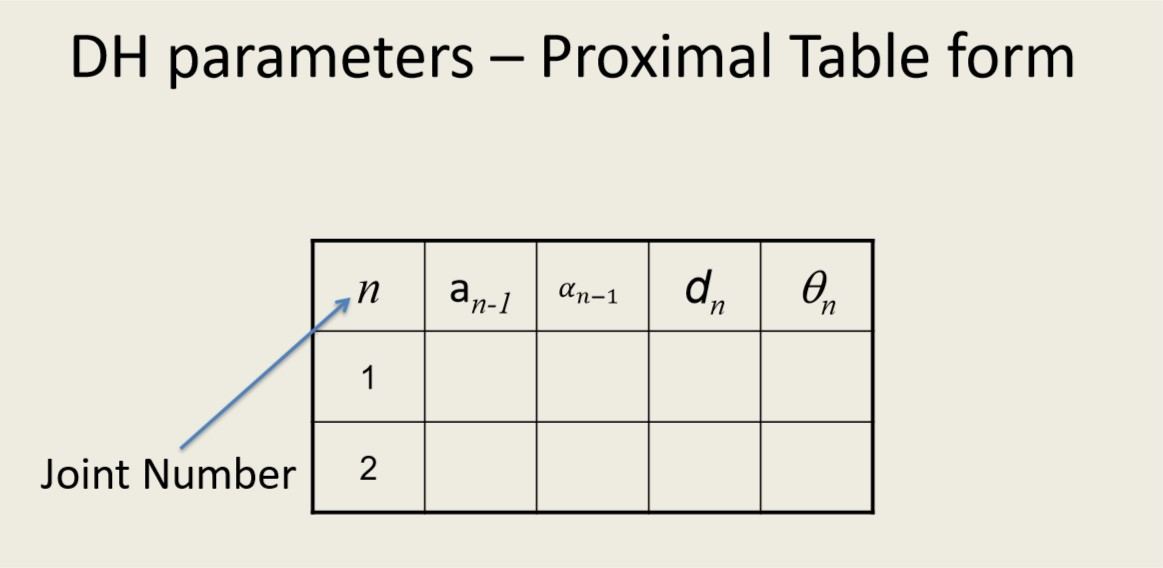The homogeneous transformation from link i-1 to link i is represented as a product of four basic transformations as follows: \begin{equation} \begin{aligned} ^{i-1}T_i&= R(x_{i-1},\alpha_{i-1})T(x_{i-1},a_{i-1})R(z_i,\theta_i)T(z_i,d_i)\\ &= \left[ \begin{matrix} 1& 0 &0 &0\\ 0& cos\alpha_{i-1} &-sin\alpha_{i-1} &0\\ 0& sin\alpha_{i-1}& cos\alpha_{i-1}& 0\\ 0& 0& 0& 1 \end{matrix} \right] \left[ \begin{matrix} 1& 0 &0 &a_{i-1}\\ 0& 1 &0 &0\\ 0& 0& 1& 0\\ 0& 0& 0& 1 \end{matrix} \right] \left[ \begin{matrix} cos\theta_i& -sin\theta_i& 0& 0\\ sin\theta_i& cos\theta_i& 0& 0\\ 0& 0& 0& 0\\ 0& 0& 0& 1 \end{matrix} \right] \left[ \begin{matrix} 1& 0 &0 &0\\ 0&1& 0 &0 \\ 0& 0& 1& d_i\\ 0& 0& 0& 1 \end{matrix} \right]\\ &= \left[ \begin{matrix} cos\theta_i& -sin\theta_i& 0& a_{i-1}\\ sin\theta_icos\alpha_{i-1}& cos\theta_icos\alpha_{i-1}& -sin\alpha_{i-1}& -d_isin\alpha_{i-1}\\ sin\theta_isin\alpha_{i-1}& cos\theta_isin\alpha_{i-1}& cos\alpha_{i-1}& d_icos\alpha_{i-1}\\ 0& 0& 0& 1 \end{matrix} \right] \end{aligned} \end{equation}

### Algorithm for Modified Convention

1. Assigning of base frame: link 0
• $a_0=0$
• $\alpha_0=0$
• $d_1=0$ if revolute joint
• $\theta_1=0$ if prismatic joint
• Identify links and name the frames by number according to the link they are attach to
• Identify joints. Link i has two joints axes: $z_i$ of joint i and $z_{i+1}$ of joint i+1
2. Identify the common normal between $z_i$ and $z_{i+1}$, or the origin point of intersection of common normal $a_i$ with $z_i$ axis
3. Assign the $z_i$ axis along the i-th joint axis
4. Assign $x_i$ axis along the common normal $a_i$ in the direction from $z_i$ to $z_{i+1}$. In the case of $a_i=0$, $x_i$ is normal to the plane of $z_i$ and $z_{i+1}$ axes
• if two z axes are parallel, pick the common normal that is collinear with the common normal of the previous joint
• If the z-axes are intersecting, we assign the x-axis along a line perpendicular to the plane formed by the two axes
5. Assign $y_i$ based on right-hand rule
6. End-effector frame assigning.
• if joint n is revolute
• $x_n$ if along $x_{n-1}$ when $\theta_n=0$
• origin n is chosen so that $d_n=0$
• if joint n is prismatic
• $x_n$ is chosen so that $\theta_n=0$
• origin n is chosen at the intersection of $x_{n-1}$ with $z_n$ so that $d_n=0$